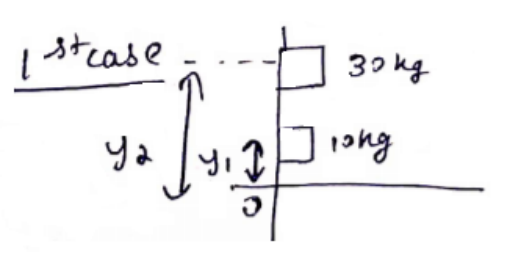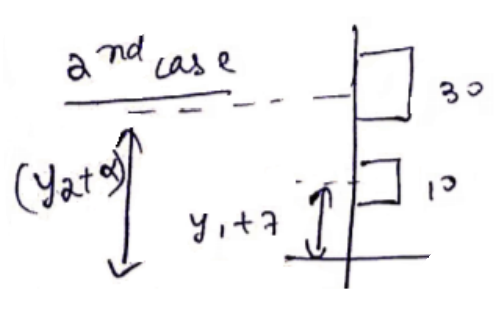# Solve the following :

Question:

Two blocks of masses $10 \mathrm{Kg}$ and $30 \mathrm{Kg}$ are placed along a vertical line. The first block is raised through a height of $7 \mathrm{~cm}$. By what distance should the second mass be moved to raise the center of mass by $1 \mathrm{~cm}$ ?

Solution:$y_{\text {COM }}=\frac{30 y_{2}+10 y_{1}}{40}$$y_{\text {COM }}^{\prime}=\frac{10\left(y_{1}+7\right)+30\left(y_{2}+\alpha\right)}{40}$

$1+y_{C O M}=y_{C O M}^{\prime}$

$\Rightarrow 40=70+30 \alpha$

$\Rightarrow \alpha=\frac{-30}{30}=-1 \mathrm{~cm}$

$y_{2}$ should be moved $1 \mathrm{~cm}$ downward.